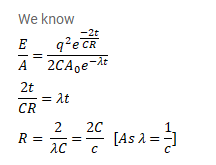# A charged capacitor of capacitance C is discharged through a resistance R.

Question:

A charged capacitor of capacitance $\mathrm{C}$ is discharged through a resistance $\mathrm{R}$. A radioactive sample decays with an averagelife. Find the value of $\mathrm{R}$ for which the ratio of electrostatic field energy stored in the capacitor to the activity of the radioactive sample remains constant in time.

Solution: